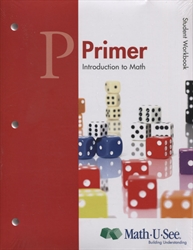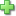# Math-U-See Primer - Student Workbook

## Introduction to Math

Publisher: Math-U-See
Consumable Workbook
Price: \$40.00

See series description for full review.

Focus: Have Fun Introducing Math

Prerequisite: The student is beginning to count and quantify.

The Primer level is an introduction to “doing math.” The emphasis of the Primer level is not for the students to demonstrate mastery of concepts and skills but to become familiar with them and ease into formal learning. Students will learn how to write numerals and basic counting. Additionally, students will be introduced to addition and subtraction, skip counting, geometric shapes, and telling time. They will become familiar with the Math-U-See Manipulatives and develop a positive attitude towards learning math.

Lessons

1. Number Recognition
2. Writing Numerals
3. Number Recognition and Writing Numerals
4. Geometric Shapes: Rectangles
5. Number Recognition and Writing Numerals
6. Geometric Shapes: Circles
7. Number Recognition and Writing Numerals
8. Geometric Shapes: Triangles
9. Place Value: Units and Tens
10. Place Value: Hundreds
11. Unit Bars
14. Counting to 20
16. Shapes: Squares; Addition: 4 + 4 and 5 + 5
17. Skip Counting by Two
19. Skip Counting by 10
21. Solving for an Unknown
22. Skip Counting by Five
23. Tally Marks
25. Skip Count to Find Area
26. Telling Time with Minutes
27. Telling Time with Hours
28. Telling Time with Minutes and Hours
29. Subtraction: Introduction and Symbol
30. Subtraction: –1

## Sample Lesson

Did you find this review helpful?
Series DescriptionClick here to write a review